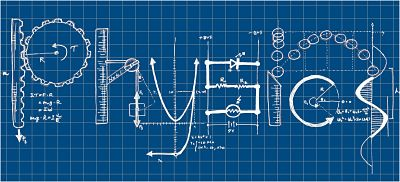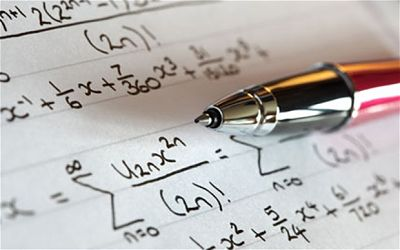With IIT-JEE exam just a few days away, students are being dragged down into the deep blue storm of nervous breakdown by their own unguided preparation tactics for the exam. Obviously, this is not a good time to ponder over new preparation strategies. They should rather steer their focus on something really beyond preparationÃ¢â‚¬â€say, ways to maximize their scores in the exam.

Smart preparation gets you good scores. All right! But knowing what questions to attempt first can make a whole lot of difference. As IIT-JEE is all about accuracy, speed, and knowing the questions you should do first, you can never skip any one of the above aspects if you are looking to score well. But the catch is that your speed and accuracy in the exam depends a lot on questions you attempt first.

Considering this theory, we have brought you a detailed subject-wise study of questions you must attempt first to score well in IIT-JEE. So here we go:

PhysicsWe already know that IIT-JEE is not entirely about solving all the problems. The success in this exam lies in the number of questions answered by students in a certain period. Hence, it is all about accuracy and speed.

So once you are done with Mathematics and Chemistry section, take a break for 2 minutes to regain your level of concentration.Ã‚Â  Obviously, you cannot solve all the problems manually in such a sort span period of time. If you try to solve all the problems in one go, then you may not even come close to many questions at the end of the test. To deal with this problem, you got to improve your IQ level.

Questions from electricity and magnetism are very easy to attempt. If you know correct formulas and concepts regarding the direction of electric and magnetic field (Like Application of Gauss law, application of Biot-Savart law, Faraday ‘s law, right hand and left thumb rule for current, electric field and magnetic field direction) then you can sort out at least two options from four choices. This way you have minimum choices to solve out of four.

You must find an easy way to get the right option, which will also save you time for other questions. Around 20 – 25% of questions are from this section. Ã‚Â Another part that students must give priority to is modern physics (atomic physics, nuclear physics, and special theory of relativity). There are a number of regular questions from this section. This part has 10 – 15% questions. You can solve questions from this section using direct formula, which also saves you time. Some problems in JEE, you can also sort out the options by using Dimensional analysis and units. This way you save your time and use it at the end for difficult problems.

You must not attempt questions serial wise. Have a look at the question paper and attempt those appear easy to you. Start with two-line questions or questions that require direct formulas. You should slowly come to moderate level questions. You will not only solve difficult questions at the end, but also get enough time to review your answers. If you try a particular question randomly from the test paper, you may or may not find the answer if its level is difficult. Even if you finally answer it, you have taken too much time to answer it.

MathematicsMathematics is the most scoring section of JEE. But time management is extremely important. You must first attempt the questions you are most confident about. Say, while solving sample papers if you could attempt the questions of algebra easily and accurately, then you must begin with algebra. But the questions which are lengthy or involve too many calculations should not be attempted in the beginning. Begin with matrices, quadratic equations or coordinate geometry. In questions of conic sections, first try to identify the type of conic section i.e. whether it is parabola, hyperbola or ellipse and then try to apply the properties. Circles and straight lines often fetch some direct questions.

JEE is all about time management. Aspirants must try to solve questions using various tricks so as to save time. Hence, smart work can actually help in obtaining correct solutions in less time. Matrices and determinants is a scoring topic of mathematics syllabus of JEE. It is important to know the various types of matrices and their properties. Like if the question asks about the rank of matrix then the matrix should be converted to echelon form and then the rank can be computed easily. Some questions involve multiplication of matrices, but instead of carrying out the complete multiplication, try to compute a particular row or column which could help you in eliminating the options. Trace of matrix gives the sum of roots and determinant gives the product of roots. Hence these facts also help in solving various questions.

Quadratic Equations is an important topic of IIT JEE. It is a simple topic which can fetch good marks.Ã‚Â  It is important to know the formulae of sum and product of roots. Whenever there is a question on quadratic equation, one should first calculate the sum and product of roots. Most of the questions can be solved using these two values. Secondly, at times there are questions on solution of an equation. In that case, one should try to substitute the options in the given equation; the values which satisfy the equation are the solutions. Hence, instead of attempting to solve the question and computing the solutions, the options should be substituted in the question. This saves time and helps in reaching at the solution easily.

ChemistryUndoubtedly, students start with questions they have good command over. But still there are a few ideal points, which will help them in deciding the order of questions to be answered.

• Students should avoid answering questions of organic chemistry in the very beginning as these questions are tricky and confusing.
• It is always a good idea to start withÃ‚Â  questions of inorganic chemistry from the following chapters:
1. P- block elements
2. S- block elements
3. Classification of elements andÃ‚Â  periodicity in property:

Questions from these chapters are mostly direct and based on the direct knowledge of the concepts.

Example:
Which of the following arrangements does not represents the correct order of the property stated against it?Ã‚Â Ã‚Â Ã‚Â Ã‚Â  (IIT (MAIN)-2013

1. Ni2+ < Co2+ < Fe2+ <Mn2+ : Ionic size
2. O3+ < Fe3+ < Cr3+ < Sc3+ : Stability in aqueous solutions
3. Sc < Ti < Cr < Mn : number of oxidation states
4. V2+ <Cr2+ <Mn2+ <Fe2+ : Paramagnetic behavior
• Some topics are interlinked to each other. It is always a good idea to solve questions of these chapters/topics.

1. Mole concept , Solutions and gaseous state
2. Redox reactions, electrochemistry and ionic equilibrium.
3. Haloalkanes and Haloarenes; Alcohol phenoles and ethers ; Aldehyde, ketones and carboxylic acids;
4. P- block elements , s-block elements and Classification of elements and periodicity in properties
• Order of the answering ofÃ‚Â  questions should be
1. Inorganic chemistry
2. Physical chemistry
3. Organic chemistry
• DonÃ¢â‚¬â„¢t waste too much time on a single question. If you feel that a question is taking too much time just move to the other one because you have to answer so many question in very limited time.